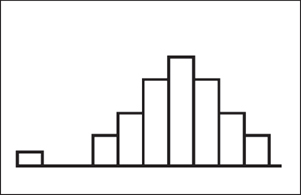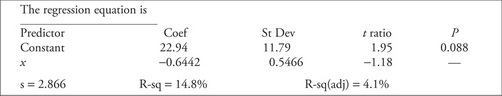# AP Statistics Practice Test 9

### Test Information10 questions23 minutes

1. Eighteen trials of a binomial random variable Xare conducted. If the probability of success for any one trial is 0.4, write the mathematical expression you would need to evaluate to find P(X = 7). Do not evaluate.

2. Two variables, xand y, seem to be exponentially related. The natural logarithm of each yvalue is taken and the least-squares regression line of ln(y) on xis determined to be ln(y) = 3.2 + 0.42x. What is the predicted value of ywhen x= 7?

3. You need to construct a 94% confidence interval for a population proportion. What is the upper critical value of zto be used in constructing this interval?

4.Which of the following best describes the shape of the histogram at the left?

5. The probability is 0.2 that a value selected at random from a normal distribution with mean 600 and standard deviation 15 will be above what number?

6. Which of the following are examples of continuous data?

I. The speed your car goes

II. The number of outcomes of a binomial experiment

III. The average temperature in San Francisco

IV. The wingspan of a bird

V. The jersey numbers of a football team

7. Use the following computer output for a least-squares regression for Questions below.What is the equation of the least-squares regression line?

8. Use the following computer output for a least-squares regression for Questions below.Given that the analysis is based on 10 datapoints, what is the P-value for the t-test of the hypothesis H0: β= 0 versus HA: β≠ 0?

9. "A hypothesis test yields a P-value of 0.20." Which of the following best describes what is meant by this statement?

10. A random sample of 25 men and a separate random sample of 25 women are selected to answer questions about attitudes toward abortion. The answers were categorized as "pro-life" or "pro-choice." Which of the following is the proper null hypothesis for this situation?